# Complexity reduction techniques with gene expression data

This tutorial will focus on different reduction complex techniques using gene expression data. In this tutorial and in the following I will use data from acute myeloid leukemia (AML), which is one of the most fatal malignancies. Genomic information and transcriptome information have already led to rediscuss the classification of AML. Indeed, leukemia malignancies show specific signature that allow to subclassify them. This is important to identify patient subset and better tailor therapeutic option, identify new potential target and predictive models. Primary diagnosis (which relies on immunophenotyping, morphology analysis) is expensive, requires experts and specific infrastructure. On the contrary, AI or machine learning approach can potentially low the cost (which is specifically important in low GDP countries).

On acute myeloid leukemia: here

On medical image analysis an AI: here

On artificial intelligence on biological data: here

Dataset is available here: dataset

The dataset used in this tutorial is obtained from Warnat-Herresthal et al. (1)They collected and re-analyzed many datasets from leukemia. In their article, they divided in three datasets (according to the microarray platform). They included in the database only human samples of three specific microarray platform. In this tutorial for convenience, I will use the first dataset.

Microarray technique was developed in the early 90’ with the aim to speed up drug development. Microarray essentially is a photolithography technique where thousands of DNA probes are spotted in a chip. The complementary DNA strand is binding on the probe DNA and this binding is measured. Considering the possibility of measure thousands of genes at one shot, many efforts were spent in developing bioinformatic techniques to analyze the data.

Microarray allows scientist to measure the mRNA expression of thousands of genes in a single shot. In most of the case, the dataset is composed by a small number of observations and high number of features. For example, a dataset is showing around 2000 samples (observations, m) and around 14000 genes (features, n). In average while datasets are around one hundred sample, they contain thousands of genes, where is common that m is far more exceeding n. This has led to the need of dimensional reduction techniques and other tools to retrieve significant knowledge hidden in the data (2). Indeed, this unbalance (m x n) is affecting machine learning model trained on the microarray dataset. Many genes are poorly expressed (invariant along the dataset) or redundant, considering as variables inside the model is just computationally expensive without any gain. Dimensional reduction techniques can be useful for feature selection (find relevant features in the dataset while discarding the others), clustering and a quick glance to the data.

I have assumed you already have imported the dataset on google drive.

`%reload_ext autoreload%autoreload 2%matplotlib inlinefrom google.colab import drivedrive.mount(‘/content/gdrive’, force_remount=True)`

import necessary libraries and the dataset:

`#import necessary libraryimport numpy as npimport pandas as pdimport matplotlib.pyplot as pltimport seaborn as snsimport umap#datasetdata = pd.read_table("/content/gdrive/My Drive/aml/201028_GSE122505_Leukemia_clean.txt", sep = "\t")`

After loading the dataset and necessary library let’s give a glance on the number of available disease conditions in the dataset.

`#table of the diseasedata.disease.value_counts()`

There are two category that are dominating the dataset: Acute Myeloid Leukemia (AML) and Acute Lymphoid Leukemia (ALL). For easier visualization we are grouping some categories.

`#removing some disease typedata["disease"] = np.where(data["disease"] == "Diabetes_Type_I" , "Diabetes", data["disease"])data["disease"] = np.where(data["disease"] == "Diabetes_Type_II" , "Diabetes", data["disease"])other = ['CML','clinically_isolated_syndrome', 'MDS', 'DS_transient_myeloproliferative_disorder']data = data[~data.disease.isin(other)]data.shapetarget = data["disease"]df = data.drop("disease", 1)df = df.drop("GSM", 1)df = df.drop("FAB", 1)df.shapetarget.value_counts()`

We are also filter out the features with low variance and scaling the remaining features.

`df = df.drop(df.var()[(df.var() < 0.3)].index, axis=1)from scipy.stats import zscoredf = df.apply(zscore)df.shape`

We are also filter out the features with low variance and scaling the remaining features.

`df = df.drop(df.var()[(df.var() < 0.3)].index, axis=1)from scipy.stats import zscoredf = df.apply(zscore)df.shape`

I will show different techniques in this tutorial, starting from Principal Component Analysis (PCA). PCA is an unsupervised linear dimensionality reduction and data visualization technique and one the most used. PCA is used for highly dimensional data transforming the original set of features in a new set of vectors (principal components).

We are choosing the number of components we are interested (we are selecting 2 since we are interested to data visualization in this context). In other cases, we can select more components especially for feature selection or clustering. PCA is preserving the total variance, we can print how much of the variance the two components are explaining.

`from sklearn.decomposition import PCApca = PCA(n_components=2)X = pca.fit(df).transform(df)print(pca.explained_variance_ratio_)`

we will use seaborn library to visualize the data. We are encoding the disease categories using LabelEncoder, since character variables are not suitable in many machine learning algorithms. We are also creating a dataframe and saving it, if we interested to keep the results.

`from sklearn.preprocessing import LabelEncoderle = LabelEncoder()le.fit(target)y_lan = le.transform(target)pca_df = pd.DataFrame(columns = [“x”, “y”, “name”, “label”])pca_df[“PCA1”] = X[:, 0]pca_df[“PCA2”] = X[:, 1]pca_df[“Disease”] = targetpca_df[“label”] = y_lansns.set(style=”whitegrid”, palette=”muted”)#sns.set_theme(style=”whitegrid”)ax = sns.scatterplot(x=”PCA1", y=”PCA2", hue=”Disease”, data=pca_df)# Put the legend out of the figureplt.legend(bbox_to_anchor=(1.05, 1), loc=2, borderaxespad=0.)#saving the graph in png or jpeg#plt.savefig(“GSE122505_Leukemia_PCA.pdf”, dpi = 300)#plt.savefig(“GSE122505_Leukemia_PCA.png”)#pca_df.to_csv(“GSE122505_Leukemia_PCA.csv”)`

t-distributed stochastic neighbourhood embedding (t-SNE) is also an unsupervised non-linear technique used for dimensionality reduction and visualization, where the main idea is to preserve the neighborhood of two point from a high dimensional space to a low dimensional space. t-SNE is a flexible technique but requires hyper-parameters tuning contrarily to PCA. Since we are interested to visualize the data, we are selecting 2 components. The choose of the hyper-parameters is heavily influencing the results, check this great tutorial about hyper-parameter choice and t-SNE result interpretation.

As a rule of thumb that you can follow to set hyper-parameters:

• the perplexity should be around N ^ (1/2), where N is the number of samples. Default perplexity is often considered 30, and other researcher suggest 1% of sample size as maximum perplexity to use. Perplexity is one of the main parameters, it could be view as a balance between the preservation of global and local structure.
• The number of iterations, in average 1000–2000 interaction should be fine. Few interactions would not allow cluster formation and too many iterations are computationally too long. An empirical law from t-SNE graph observation suggests that when the largest distance between data points is around 100, the algorithm reached convergence.
• Learning rate is in the range of 10 to 1000, too high and data are too spread, too low and the data are too compressed. Default learning rate in many libraries is set to 100 or 200, some authors suggests to set learning rate as N/12 if the results is over 200
`from sklearn.manifold import TSNEtsne = TSNE(n_components=2, perplexity=48.0, learning_rate=200.0, n_iter=2000 )X = tsne.fit_transform(df, y_lan)tsne_df = pd.DataFrame(columns = ["x", "y", "name", "label"])tsne_df["tSNE1"] = X[:, 0]tsne_df["tSNE2"] = X[:, 1]tsne_df["Disease"] = targettsne_df["label"] = y_lansns.set(style="whitegrid", palette="muted")ax = sns.scatterplot(x="tSNE1", y="tSNE2", hue="Disease",  data=tsne_df)# Put the legend out of the figureplt.legend(bbox_to_anchor=(1.05, 1), loc=2, borderaxespad=0.)#plt.savefig("GSE122505_Leukemia_tSNE.pdf", dpi = 300)#plt.savefig("GSE122505_Leukemia_tSNE.png")#tsne_df.to_csv("GSE122505_Leukemia_tSNE.csv")`

t-SNE can have difficulties to such high dimension number, so many researchers suggest to reduce the dimensionality. For instance, you can obtain this using PCA before apply t-SNE. In this case, we use the PCA and we are retaining the first 50 components. On these50 components we are running the t-SNE.

`pca = PCA(n_components=50)X_pca = pca.fit(df).transform(df)tsne = TSNE(n_components=2, perplexity=48.0, learning_rate=200.0, n_iter=2000 )X = tsne.fit_transform(X_pca, y_lan)tsne_df = pd.DataFrame(columns = ["x", "y", "name", "label"])tsne_df["tSNE1"] = X[:, 0]tsne_df["tSNE2"] = X[:, 1]tsne_df["Disease"] = targettsne_df["label"] = y_lansns.set(style="whitegrid", palette="muted")ax = sns.scatterplot(x="tSNE1", y="tSNE2", hue="Disease",  data=tsne_df)# Put the legend out of the figureplt.legend(bbox_to_anchor=(1.05, 1), loc=2, borderaxespad=0.)#plt.savefig("GSE122505_Leukemia_PCA_tSNE.pdf", dpi = 300)#plt.savefig("GSE122505_Leukemia_PCA_tSNE.png")#tsne_df.to_csv("GSE122505_Leukemia_PCA_tSNE.csv")`

Differences between PCA and t-SNE:

• PCA is a linear dimension technique while t-SNE is not linear.
• PCA tries to preserve the global structure of data while t-SNE tries to preserve the local structure.
• PCA do not require to select hyper-parameters while t-SNE yes.
• t-SNE can handle outliers while PCA is highly affected.
• PCA is a deterministic algorithm while t-SNE is randomized
• PCA is rotating the vectors, preserving the variance. t-SNE instead is minimizing the distance between two points in the gaussian.

Based on this, one advantage for PCA is that is a deterministic algorithm, while t-SNE varies on each run since it is randomized. The t-SNE algorithm can have different local minima that can change the visualization due to different solutions (this is due to the fact that the cost function is not convex and thus with different initializations you can obtain different results) which turn in a lower reproducibility. PCA component can be interpreted, we can use for feature selection and we identify which components represent the maximum variance. PCA is suitable to project unseen data since the eigenvectors are a new axis system we can use for new data projection. PCA and t-SNE are not dealing with incomplete data, but PCA-derived algorithms are now able to do it (in this case the PCA followed by t-SNE is a solution).

t-SNE visualization are indeed in average superior to PCA visualization. Moreover, it is preferred to transcriptomic data, since it try to preserve local structures. t-SNE is generating a plot with different clusters, which are often in good agreement with clusters that are obtained by other clustering algorithm (3). However, t-SNE itself has some limitation: it is not preserving the global structures (inter-cluster relationship), it is computationally expensive and is not very meaningfully when approaching very large datasets (4).

In the last years a new technique has been extensively using in the context of omic data visualization (often for single cell RNA seq). uniform manifold approximation and projection (UMAP) has been claimed to be superior to t-SNE in preserving local and global data structure and to be at the same time a faster algorithm (4,5). Interestingly, both t-SNE and UMAP show a strong local clustering but similar clusters in UMAP are closer (global structure). As an example, in the fashion MNIST, similar images (like sweaters or coats) are clustering with both algorithms but in UMAP similar categories are co-locating (like sweaters, shirts). This is nicely showed here.

In a simplistic view, UMAP build a high-dimensional connected graph (where the edge weight between two point is the likelihood that these two points are connected) and then it optimes that graph to a lower dimensional projection.

Selecting the right parameters value ensure the balance between local and global projection:

• Number of neighbors: the number of approximate nearest neighbors to a point is used by UMAP to construct the high dimensional graph. A low value is directing UMAP towards more attention to the local structure, while a high value is directing the algorithm to the global structure losing the fine details.
• Minimum distance: it is controlling the minimum distance between points in the lower dimensional space, in the clusters. Selecting a low value leads to tightly packed embeddings.
• Number of components: you can select more than a component that can be useful if you are using UMAP for clustering or if you using UMAP as a step in a machine learning algorithm.
• Metric: you can select which metric is used to compute the distance between the points. Euclidean distance is the most used.

However, UMAP is also a not-deterministic algorithm and interpretability of axis and distance has no meaning (not like in PCA). You may need more than a plot, since is stochastic using the same parameter values will lead to different visualization. Moreover, the choosing of the parameter value are affecting the visualization, so it is best to try different combination. Clustering on original data is limited by the curse of dimensionality (m x n unbalance I aforementioned), while is quite complicate on t-SNE it is agile to do on UMAP and PCA results (which it will be deepen in a following tutorial).

`import umapreducer = umap.UMAP(n_neighbors =  100, min_dist= 0.2, metric ="euclidean")X = reducer.fit_transform(df)umap_df = pd.DataFrame(columns = ["x", "y", "name", "label"])umap_df["UMAP1"] = X[:, 0]umap_df["UMAP2"] = X[:, 1]umap_df["Disease"] = targetumap_df["label"] = y_lansns.set(style="whitegrid", palette="muted")ax = sns.scatterplot(x="UMAP1", y="UMAP2", hue="Disease",  data=umap_df)# Put the legend out of the figureplt.legend(bbox_to_anchor=(1.05, 1), loc=2, borderaxespad=0.)#plt.savefig("GSE122505_Leukemia_UMAP.pdf", dpi = 300)#plt.savefig("GSE122505_Leukemia_UMAP.png")#ctrl_df.to_csv("GSE122505_Leukemia_UMAP.csv")`

In the same way, we can first use PCA and then run a UMAP.

`pca = PCA(n_components=50)X_pca = pca.fit(df).transform(df)reducer = umap.UMAP(n_neighbors =  100, min_dist= 0.2, metric ="euclidean")X = reducer.fit_transform(X_pca)umap_df = pd.DataFrame(columns = ["x", "y", "name", "label"])umap_df["UMAP1"] = X[:, 0]umap_df["UMAP2"] = X[:, 1]umap_df["Disease"] = targetumap_df["label"] = y_lansns.set(style="whitegrid", palette="muted")ax = sns.scatterplot(x="UMAP1", y="UMAP2", hue="Disease",  data=umap_df)# Put the legend out of the figureplt.legend(bbox_to_anchor=(1.05, 1), loc=2, borderaxespad=0.)#plt.savefig("GSE122505_Leukemia_PCA_UMAP.pdf", dpi = 300)#plt.savefig("GSE122505_Leukemia_PCA_UMAP.png")#ctrl_df.to_csv("GSE122505_Leukemia_PCA_UMAP.csv")`

The results are similar in both cases.

We can then use the UMAP for different exploratory analysis. On the UMAP projection we can highlight a particular gene, in this case ANXA1.

`umap_df["ANXA1"] = df["ANXA1"]sns.set(style="whitegrid", palette="muted")ax = sns.scatterplot(x="UMAP1", y="UMAP2", hue="ANXA1",  data=umap_df)# Put the legend out of the figureplt.legend(bbox_to_anchor=(1.05, 1), loc=2, borderaxespad=0.)`

There are also other dimension reduction techniques but they will presented in following tutorials.

Selected Bibliography

1. Musheer R, Verma CK, Srivastava N. Dimension reduction methods for microarray data: a review. AIMS Bioengineering. 2017;4:179–97.

2. Warnat-Herresthal S, Perrakis K, Taschler B, Becker M, Baßler K, Beyer M, et al. Scalable Prediction of Acute Myeloid Leukemia Using High-Dimensional Machine Learning and Blood Transcriptomics. iScience [Internet]. 2019 [cited 2020 Oct 26];23. Available from: https://www.ncbi.nlm.nih.gov/pmc/articles/PMC6992905/

3. Kobak D, Berens P. The art of using t-SNE for single-cell transcriptomics. Nature Communications. Nature Publishing Group; 2019;10:5416.

4. Becht E, McInnes L, Healy J, Dutertre C-A, Kwok IWH, Ng LG, et al. Dimensionality reduction for visualizing single-cell data using UMAP. Nature Biotechnology. Nature Publishing Group; 2019;37:38–44.

5. McInnes L, Healy J, Melville J. UMAP: Uniform Manifold Approximation and Projection for Dimension Reduction. arXiv:180203426 [cs, stat] [Internet]. 2020 [cited 2020 Oct 28]; Available from: http://arxiv.org/abs/1802.03426

# Code availability

You find the code for the tutorial and all the images here. You can use the provided Colab notebook without the need to have any package installed on your pc.

# if you have found it interesting:

Please share it, you can also subscribe to get notified when I publish articles, you can also connect or reach me on LinkedIn. Thanks for your support!

--

--

--

## More from Peter Moss Leukaemia MedTech Research CIC

Researching into AI & modern technologies, and how they can be used in the fight against Leukemia.

## Gosloto 6–45 Morning Results: Saturday 05 March 2022## Digitizing a Century of History with Data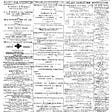## Basics of Kernels and Convolutions with OpenCV## Python Programming…in R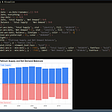## Working with Multi-Lingual data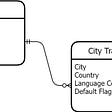## RecSys16: Adaptive, Personalized Diversity for Visual Discovery## 4 Ways To Improve Your Plotly Graphs## Salvatore Raieli

Senior data scientist, passionate and writing about bioinformatics, machine learning, and AI. https://www.linkedin.com/in/salvatore-raieli/

## Ames Housing Price Predic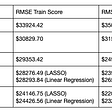## KNN Algorithm for Classification and Regression: Hands-On With Scikit- Learn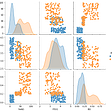## Data Science Project: Sales Forecasting with Arima## Storytelling with Data — A Key Skill for Data Scientists